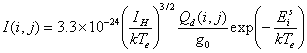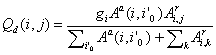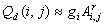How to estimate intensity of dielectronic satellite lines

Intensity of a dielectronic satellite line can be calculated with a following equation:(photons cm3s-1),

where IH is the ionization potential of hydrogen, Te is electron temperature, g0 is the statistical weight of initial parent state i0, EiS is energy level of autoionizing state i measured from initial state i0, and Qd(i,j) is intensity factor obtained as follows with gi statistical weight of autoionizing state i, Aa autoionization rate, and Ar radiative transition probability:.

For the most cases, Aa is much larger than Ar and then approximately Qd becomes.

In the data set of this database, Qd and the wavelength are included as defualt, and Es (EiS in the above equation) is included in some cases.

Atomic and Molecular Data Research Center, National Institute for Fusion Science
Last updated: Sep. 28, 2002
web@dpc (+".nifs.ac.jp")